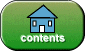revised: 09/23/99, 10/04/03, 10/19/08, 08/23/2011, 10/31/2012

#on for Loops

Instructions: For each question, choose the single best answer. Make your choice by clicking on its button. You can change your answers at any time. When the quiz is graded, the correct answers will appear in the box after each question.

1. What are the three general types of looping structures?

A.    counting loop,  sentinel-controlled loop,  and result-controlled loop.

B.    infinite loop, counting loop, nested loop

C.    while loop, for loop, do loop

D.    count up loop, count down loop, infinite loop

2. What is the output of the following program fragment?

```int j;
for ( j = 0;  j <  5; j++ )
{
System.out.print( j + " " );
}
System.out.println( );
```

A.    `1 2 3 4 5`

B.    `0 1 2 3 4`

C.    `0 1 2 3 4 5`

D.    `j j j j j `

3. What is the output of the following code fragment?

```int j;
for ( j = 10;  j >  5; j-- )
{
System.out.print( j + " " );
}
System.out.println( );
```

A.    `10 11 12 13 14 15 `

B.    `9 8 7 6 5 4 3 2 1 0`

C.    `10 9 8 7 6 5 `

D.    `10 9 8 7 6 `

4. What must the test be so that the following fragment prints out the integers 5 through and including 15?

```int j;
for ( j = 5;  ________ ; j++ )
{
System.out.print( j + " " );
}
System.out.println( );
```

A.    `j<15`

B.    `j<=16`

C.    `j<16`

D.    `j==15`

5. What must the change be so that the following fragment prints out the even integers 0 2 4 6 8 10?

```int j;
for ( j = 0; j <= 10; _______ )
System.out.print( j + " " );
System.out.println( );
```

A.    `j+2`

B.    `j = j+2`

C.    `j++++ `

D.    `++j++ `

6. What must the initialization be so that the following fragment prints out the integers -3 -2 -1 ?

```int j;
for ( _______ ; j < 0; j++    )
System.out.print( j + " " );
System.out.println( );
```

A.    `j = 0`

B.    `j < 0`

C.    `j = -3`

D.    `j = -4`

7. What is the output of the following code fragment?

```int j;
for ( j = 5;  j > -5; j-- )
System.out.print( j + " " );

System.out.println( );
```

A.    `-5 -4 -3 -2 -1 0`

B.    `5 4 3 2 1 0`

C.    `5 4 3 2 1 0 -1 -2 -3 -4 -5`

D.    `5 4 3 2 1 0 -1 -2 -3 -4`

8. What is the output of the following code fragment?

```int count = 0;
for ( ;  count < 9; ++count )
System.out.print( count + " " );

System.out.println( );
```

A.    ` 0 1 2 3 4 5 6 7 8`

B.    Nothing — the fragment will not compile.

C.    `0 1 2 3 4 5 6 7`

D.    `1 2 3 4 5 6 7 8 9 `

9. What is the output of the following code fragment?

```int count;

for ( count = 0;  count < 9;   )
{
System.out.print( count + " " );
count++ ;
}
System.out.println( );
```

A.    `0 1 2 3 4 5 6 7 8 `

B.    Nothing — the program will not compile.

C.    `count count count count count count count count count `

D.    `0 1 2 3 4 5 6 7 8 9`

10. What is the output of the following code fragment?

```int j;
for ( j = 0;  j < 5;   )
{
System.out.print( j + " " );
j++ ;
}

System.out.println( );
```

A.    ` 0 1 2 3 4 `

B.    Nothing — the program will not compile.

C.    ` 1 2 3 4 5 `

D.    ` 0 0 0 0 0 0 0 0 0 0 0 0 0 0 0 0 0 0 0 0 0 0 0 0 0 0 0 ... `

The number you got right:       Percent Correct:       Letter Grade:If you have returned here from another page, or have re-loaded this page, you will need to click again on each of your choices for the grading program to work correctly.